﻿ 三维移动脉动源非定常波系演变与试验验证

# 三维移动脉动源非定常波系演变与试验验证Unsteady Wave Evolution and Test Verification for Three-Dimensional Translating-Pulsating Source Green Function

Abstract: In order to investigate the characteristics of unsteady wave patterns for three-dimension trans-lating-pulsating source Green function, a method with the combination of theoretical analysis and test verification is proposed in present paper. By adopting stationary-phase analysis method, the parametric equations of stationary-phase curves for far-field waves of Bessho-type translat-ing-pulsating source Green function are derived. In the framework of frequency-domain theory, the steady shapes of unsteady wave patterns are obtained on the free surface. For the unsteady wave patterns such as ring-fan wave and ring wave, fan wave and outer-V wave and inner-V wave, the evolution laws of wave-pattern behavior are analyzed in detail. Based on the towing-tank test of two ship models advancing parallel in waves, wetness phenomena on the shipboard for a ship model is captured in typical operating condition and an estimation method of the propagation region of radiation-diffraction waves is given for the advancing ship in waves. The present paper demonstrates that the unsteady wave patterns of Bessho-type translating-pulsating source Green function are inclusive of two kinds: one kind is the circular wave pattern under the consideration of forward speed effect and the other kind is the Kelvin wave pattern with the harmonic pulsating effect taken into account. And the test observation of shipboard wetness verifies the correctness of far-field wave propagation for translating-pulsating source Green function primarily.

1. 引言

Haskind型为三维移动脉动源格林函数的最初表达形式，经过简化推导后来又出现了Havelock型  、Michell型  和Bessho型  三种形式的移动脉动源格林函数。其中，Bessho型格林函数的数学表达式各项均为初等函数形式，且仅涉及单重积分，在数值计算中具有比Havelock型和Michell型更加明显的优势。然而，部分学者认为Bessho型移动脉动源格林函数的物理意义并不如上述两类格林函数明确  。分析可知，Havelock型和Michell型格林函数的近场和远场扰动项分别对应于格林函数表达式的子项，而Bessho型格林函数的近场和远场扰动项均耦合在整段积分区间内。开展Bessho型移动脉动源非定常波系的理论与试验研究不仅有利于其物理含义的明确，而且对于深化理解船舶在波浪中的扰动波特性也十分有益。

2. Bessho型移动脉动源格林函数

$G\left(p,q\right)=\frac{1}{4\text{π}}\left(\frac{1}{r}-\frac{1}{r\text{'}}\right)-\frac{\text{i}}{2\text{π}}{K}_{0}T\left(\omega \right)$ (1)

$T\left(\omega \right)={\int }_{-\text{π}+\alpha }^{-\frac{\text{π}}{\text{2}}+\phi -i\epsilon }\frac{1}{\sqrt{1+4\tau \mathrm{cos}\theta }}\left[{k}_{2}\mathrm{exp}\left({k}_{2}\omega \right)-\mathrm{sgn}c\cdot {k}_{1}\mathrm{exp}\left({k}_{1}\omega \right)\right]\text{d}\theta$

$\begin{array}{l}r\\ r\text{'}\end{array}\right\}=\sqrt{{\left(x-\xi \right)}^{2}+{\left(y-\eta \right)}^{2}+{\left(z\mp \zeta \right)}^{2}}$$\omega =Z+i\left(X\mathrm{cos}\theta +Y\mathrm{sin}\theta \right)$

$\begin{array}{l}{k}_{1}\\ {k}_{2}\end{array}\right\}=\frac{1}{2{\mathrm{cos}}^{2}\theta }\left(1+2\tau \mathrm{cos}\theta ±\sqrt{1+4\tau \mathrm{cos}\theta }\right)$${K}_{0}=g/{U}^{2}$

$\alpha =\left\{\begin{array}{cc}\begin{array}{l}{\mathrm{cos}}^{-1}\left(1/4\tau \right)\\ -i{\mathrm{cosh}}^{-1}\left(1/4\tau \right)\end{array}& \begin{array}{l}\left(4\tau >1\right)\\ \left(4\tau <1\right)\end{array}\end{array}$$\mathrm{sgn}c=sign\left[\mathrm{cos}\left(\mathrm{Re}\left(\theta \right)\right)\right]$

$\phi ={\mathrm{cos}}^{-1}\frac{X}{\sqrt{{X}^{2}+{Y}^{2}}}$$\epsilon ={\mathrm{sinh}}^{-1}\frac{|Z|}{\sqrt{{X}^{2}+{Y}^{2}}}$$\tau =U{\omega }_{e}/g$

$X={K}_{0}\left(x-\xi \right)$$Y={K}_{0}|y-\eta |$$Z={K}_{0}\left(z+\zeta \right)$

3. 同相分析法

$\Psi \left(M\right)={\left(m-\tau \right)}^{2}Z+i\cdot \left[Xm+\mathrm{sgn}s\cdot Y\sqrt{{\left(m-\tau \right)}^{4}-{m}^{2}}\right]$ (2)

$\psi \left(M\right)=Xm+\mathrm{sgn}s\cdot Y\sqrt{{\left(m-\tau \right)}^{4}-{m}^{2}}$ (3)

$\frac{\text{d}\psi }{\text{d}M}=\mathrm{sgn}c\cdot \left[X+\mathrm{sgn}s\cdot Y\frac{2{\left(m-\tau \right)}^{3}-m}{\sqrt{{\left(m-\tau \right)}^{4}-{m}^{2}}}\right]$ (4)

$\frac{X}{Y}=-\mathrm{sgn}s\cdot \frac{2{\left(m-\tau \right)}^{3}-m}{\sqrt{{\left(m-\tau \right)}^{4}-{m}^{2}}}$ (5)

$\left\{\begin{array}{l}X=\frac{2{\left(m-\tau \right)}^{3}-m}{{\left(m-\tau \right)}^{3}\left(m+\tau \right)}\psi \\ Y=-\mathrm{sgn}s\cdot \frac{\sqrt{{\left(m+\tau \right)}^{4}-{m}^{2}}}{{\left(m-\tau \right)}^{3}\left(m+\tau \right)}\psi \end{array}$ (6)

4. 移动脉动源非定常波系演变特性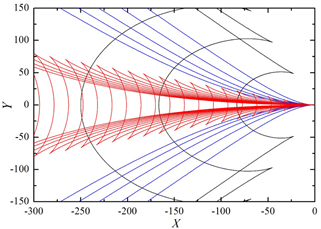(a) τ = 0.35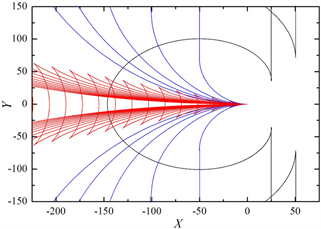(b) τ = 0.25+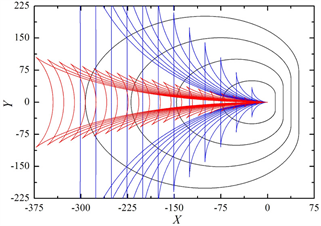(c) τ = 0.25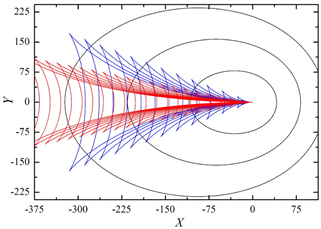(d) τ = 0.2

Figure 1. Unsteady wave patterns of Bessho form translating-pulsating source Green function versus τ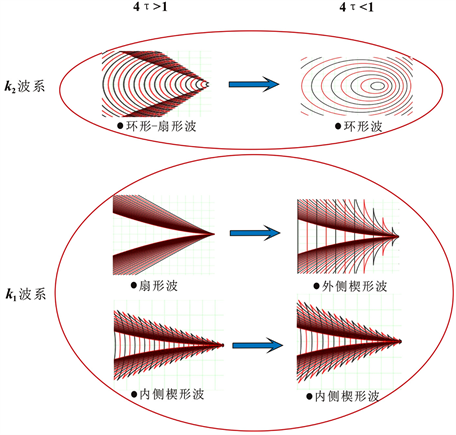Figure 2. Evolution of the unsteady wave patterns for Bessho form translating-pulsating source Green function

4.1. 环形–扇形波与环形波

k2波系中的环形–扇形波(图3)与环形波(图4)均具有显著的圆形波特征，这集中反映在点源的下游区域。上述两个波系的不同之处主要体现在：环形波的等相线为封闭式的曲线，且点源前方的环形波等相线明显比其下游稠密，这与点源具有 $ox$ 轴正向速度有关；而环形–扇形波在其波系外缘已具有散波的形态。这里，环形–扇形波的传播区域可利用该波系的边界与点源移动速度反方向的夹角来定义，该夹角称之为半楔角，显然环形波并不存在半楔角。随着参数τ的增大，环形–扇形波的半楔角逐渐变小，且波系的波长表征出减小的趋势。在参数τ = 0.25附近，点源的前方出现了环形–扇形波或环形波的横波模态，该横波的传播方向几乎与点源的速度方向平行。

4.2. 扇形波与外侧楔形波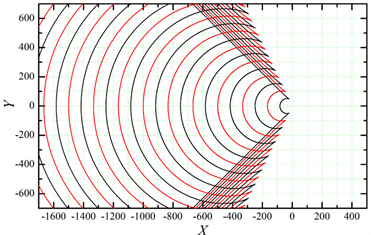(a) τ = 0.35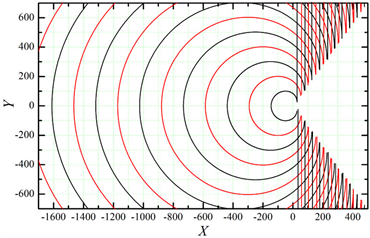(b) τ = 0.25+

Figure 3. Ring-fan wave patterns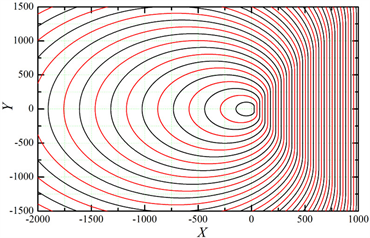(a) τ = 0.25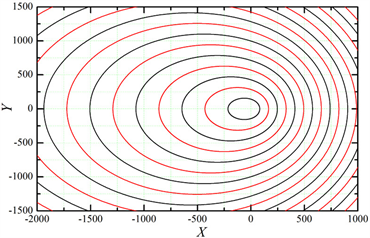(b) τ = 0.2

Figure 4. Ring wave patterns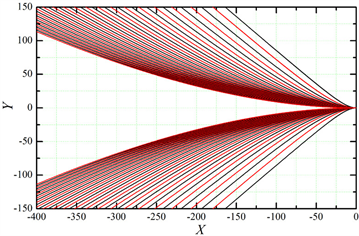(a) τ = 0.35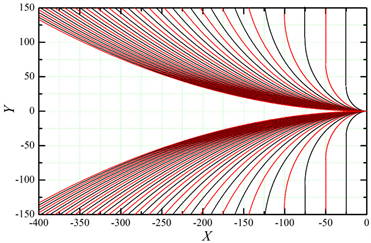(b) τ = 0.25+

Figure 5. Fan wave patterns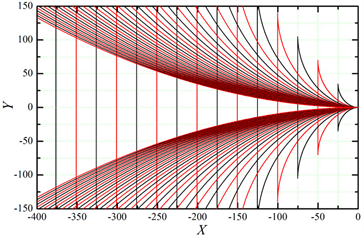(a) τ = 0.25>(b) τ = 0.2

Figure 6. Outer-V wave patterns

4.3. 内侧楔形波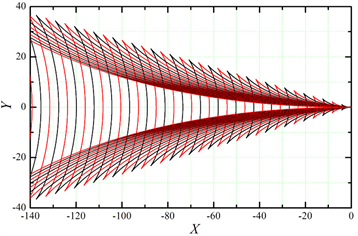(a) τ = 0.35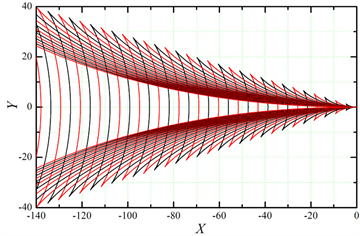(b) τ = 0.25+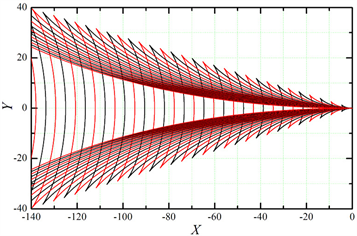(c) τ = 0.25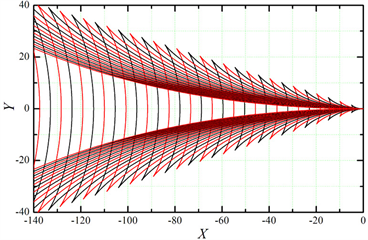(d) τ = 0.2

Figure 7. Inner-V wave patterns

5. 基于船体舷侧上浪的试验波形观测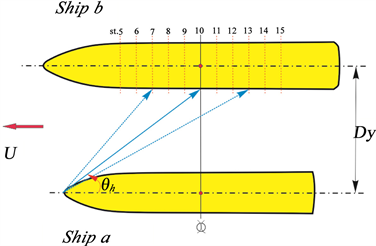Figure 8. Sketch of the wetness on the shipboard for ship b

$\mathrm{tan}{\theta }_{h}=\frac{{D}_{y}-\frac{{B}_{b}}{2}}{\frac{{L}_{a}}{2}-\frac{{L}_{b}}{20}\left(10-st.\right)}$ (7)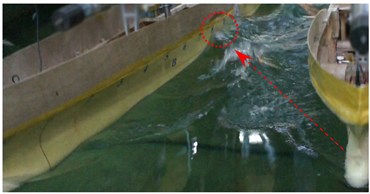(a) λ=3m, τ=0.583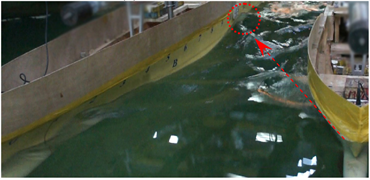(b) λ=2 m, τ=0.761

Figure 9. Typical photographs of the wetness on the shipboard for ship b at Fr = 0.153Figure 10. Experimental values of the radiation-diffraction waves for ship a and theoretical results of the ring-fan wave pattern of the translating-pulsating source

6. 结论

1) 针对Bessho型移动脉动源格林函数进行积分变换后，采用同相分析法可获得非定常波系的等相曲线族方程；随着航行速度和脉动频率的增大，移动脉动源非定常波系向后方传播的特征愈加显著。

2) 波浪中两船模并行航行的水池拖曳试验结果表明船体辐射–绕射波的传播范围与移动脉动源的环形–扇形波是基本吻合的，典型工况下的船体舷侧上浪观测初步验证了移动脉动源远场波系传播特征的正确性。

NOTES

*通讯作者。

 Haskind, M.D. (1946) The Hydrodynamic Theory of Ship Oscillations in Rolling and Pitching. Applied Mathematics and Mechanics, 10, 33-36.

 Xu, Y. and Dong, W.C. (2013) Valid Hydrodynamic Interaction Regions of Multiple Ships Advancing in Waves. Journal of Hydrodynamics, 25, 856-866.
https://doi.org/10.1016/S1001-6058(13)60433-3

 洪亮, 朱仁传, 缪国平, 等. 三维频域有航速格林函数的数值计算与分析[J]. 水动力学研究与进展A辑, 2013, 28(4): 423-430.

 Yuan, Z.M., Incecik, A. and Alexander, D. (2014) Verification of a New Radiation Condition for Two Ships Advancing in Waves. Applied Ocean Research, 48, 186-201.
https://doi.org/10.1016/j.apor.2014.08.007

 Havelock, T.H. (1958) The Effect of Speed of Advance upon the Damping of the Heave and Pitch. Transactions Institution Naval Architects, 100, 131-135.

 缪国平, 刘应中, 杨勤正, 等. 三维移动脉动源的Michell型表达[J]. 中国造船, 1995(131): 1-11.

 Bessho, M. (1977) On the Fundamental Singularity in the Theory of Ship Motions in a Seaway. Memoirs of the Defense Academy of Japan, 17, 95-105.

 Matao, T. (1992) 3D Green Function of Oscillating Body with Forward Speed on Water of Finite Depth. Journal of Kansai Society of Naval Architects of Japan, 217, 67-75.

 Yao, C.B. and Dong, W.C. (2016) Numerical Study on Local Steady Flow Effects on Hydrodynamic Interaction between Two Parallel Ships Advancing in Waves. Engineering Analysis with Boundary Elements, 66, 129-144.
https://doi.org/10.1016/j.enganabound.2016.02.007

 Fang, M.C., Yang, R.Y. and Shugan, I.V. (2011) Kelvin Ship Wake in the Wind Waves Field and on the Finite Sea Depth. Journal of Mechanics, 27, 71-77.
https://doi.org/10.1017/jmech.2011.9

Top